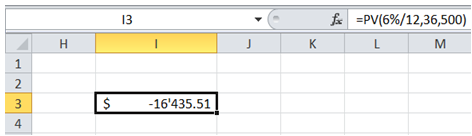## Net present value of investment in Excel

To do it in Excel, here is the answer:

a) Enter the formula =PV(6%/12,36,500) in an empty cell.

Note: First argument is interest rate - 6% is annual interest rate. Hence it is divided by 12 to get monthly rate.

Note: Second argument is number of periods - (3 years = 36 months).

Note: Third argument is payment per period - (\$500).

Above formula returns the Present Value of the investment being considered as shown below.